Chapter Notes: Gravitation

# Gravitation Chapter Notes - Science Class 9

 Table of contentsWhat is Gravitation?Free Fall or GravityMassWeightThrust and PressureArchimedes’ Principle## What is Gravitation?

Gravitation is the universal phenomenon where every object in the universe attracts every other object. This attractive force between any two objects is referred to as the "force of gravitation." Although the gravitational force is weak and typically requires substantial masses to be observed, it acts over long distances.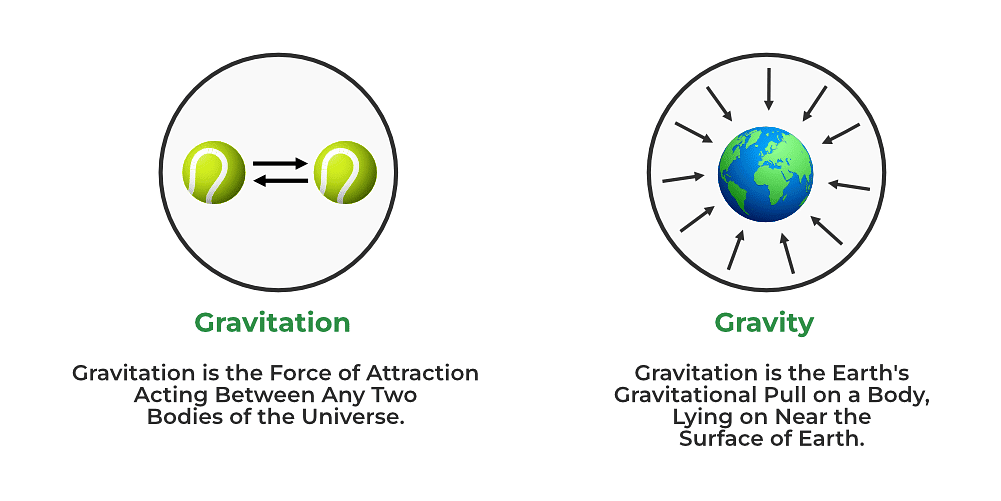Gravitation and Gravity

### Universal Law of Gravitation

According to Newton’s law of gravitation, the force of gravitational attraction between two objects is directly proportional to the product of their masses and inversely proportional to the square of the distance between them.

Question for Chapter Notes: Gravitation
Try yourself:What is the formula for the force of gravitational attraction between two objects?

If M and m be masses of two objects separated by a distance d, the gravitational force of attraction between them is given by: F = G x (mx m2) / d2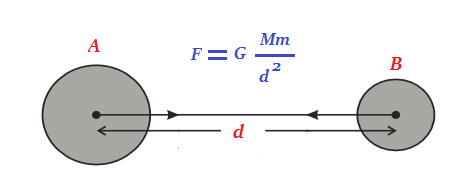where G is a constant, known as the universal constant of gravitation.

• The universal constant of gravitation G is numerically equal to the force of attraction between two objects of unit mass each separated by unit distance.
• The value of G is 6.673 x 10-11 N m2 kg-2.
• G is called a universal constant because its value does not depend on the nature of the intervening medium or temperature or any other physical variable.
• As the value of G is extremely small, the gravitational force between ordinary terrestrial objects is so small that it cannot be detected. However, the force of attraction acting on an object due to Earth, the force of attraction between Earth and the moon, and the force experienced by planets due to the gravitational attraction of Sun can be easily felt and measured.

Example 1: Suppose we have two objects: Object A with a mass of 5 kilograms and Object B with a mass of 10 kilograms. The distance between the centers of these objects is 2 meters. We'll assume the gravitational constant, G, to be approximately 6.674 × 10-11 N m2/kg2.

Solution:

Using the Universal Law of Gravitation, we can calculate the gravitational force between these objects:

F = (G * (m1 * m2)) / r2

F = (6.674 × 10-11 N m2/kg2 * (5 kg * 10 kg)) / (2 m)2

F = (6.674 × 10-11 N m2/kg* 50 kg2) / 4 m2

F = (6.674 × 10-11 N m2) / 4

F ≈ 1.6685 × 10-11 N

Therefore, the gravitational force between Object A and Object B is approximately 1.6685 × 10-11 Newtons.

Question for Chapter Notes: Gravitation
Try yourself:Which of the following pairs of objects will experience the greatest gravitational force?

### Importance Of The Universal Law Of Gravitation

The universal law of gravitation successfully explained several phenomena which were believed to be unconnected:

• The force that binds us to the Earth
• The motion of the moon around the Earth
• The motion of planets around the Sun
• The tides due to the moon and the Sun

## Free Fall or Gravity

• The force of gravitational attraction exerted by the earth on an object is called the “force of gravity’'. Force of gravity acting on an object of mass m situated on Earth’s surface or near it is given by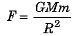where M = mass of earth and R = radius of Earth.
• The acceleration produced in a freely falling object on account of the force of gravity is known as the acceleration due to gravity. It is denoted by the symbol ‘g’.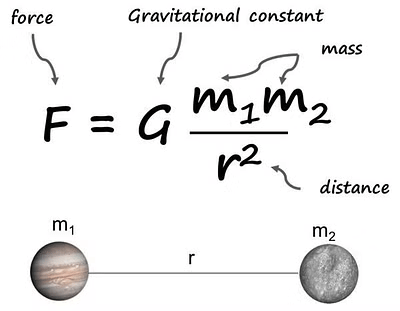Gravitation Formula

### To Calculate Value of g

• The value of acceleration due to gravity on the surface of Earth is given by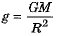The mean value of g on the surface of Earth is found to be 9.8 ms-2.
• Here's how the value of g is calculated.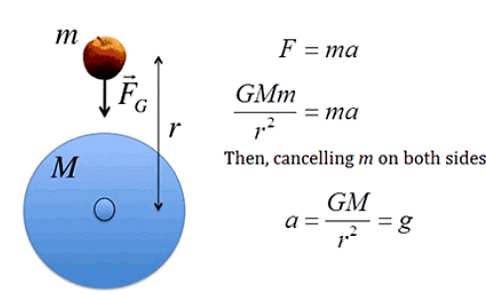Calculation of acceleration due to gravity

### Motion of Objects under the influence of Gravitational Force of the Earth

Value of g varies from place to place. On surface of earth value of g is more at poles than at the equator. Again value of g decreases as one goes away and away from the earth.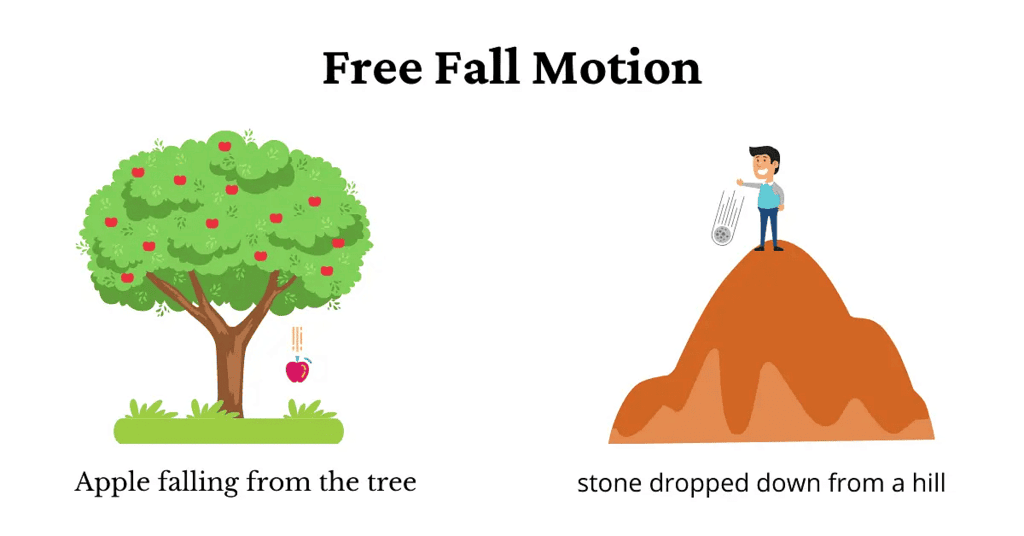Free Fall Motion

• When an object falls towards the earth under the force of gravity alone, we say that the object is in free fall. A freely falling object experiences a constant acceleration of g (=9.8ms-2) during its downward motion. However, if an object is projected vertically upward with a certain velocity, its velocity goes on decreasing due to gravity, till it comes to rest and then starts falling vertically downward under gravity.
• The three equations of motion viz, (i) v = u + at, (ii) s = ut + 1/2 at2,  and (iii) v2 - u2 = 2as are true for motion of objects under gravity. For free fall, the value of acceleration a = g = 9.8ms-2.
• If an object is just let fall from a height then in that case u = 0 and a = +g = +9.8ms-2.
• If an object is projected vertically upwards with an initial velocity u, then a = -g = -9.8ms-2 and the object will go to a maximum height h where its final velocity becomes zero (i.e. v = 0). In such a case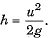Question for Chapter Notes: Gravitation
Try yourself:
Which of the following statements is true according to the universal law of gravitation?

## Mass

• The mass of an object is a measure of its inertia. Mass of an object is constant and does not change from place to place.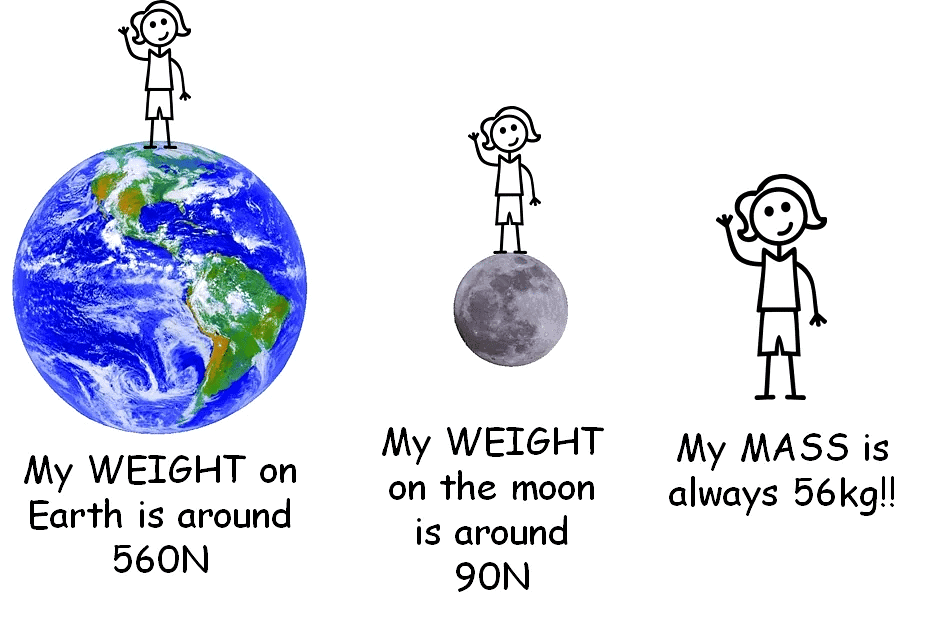Mass and Weight

## Weight

• The weight of an object is the force with which it is attracted towards the earth. Weight W of an object of mass m will be W = mg. Weight is a force acting vertically downwards. It means that it is a vector.
• As weight of an object is a force, its SI unit is newton (N).
An object of mass m = 1 kg has thus a weight of W = 1 x 9.8 = 9.8 N.
• At a given place weight of an object is directly proportional to its mass t.e.,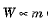(at a given place). Due to this reason at a given place, we may use the weight of an object as a measure of its mass.

### Weight of Object on the Moon

• Mass of an object remains the same everywhere but weight of an object changes from place to place. For an object of mass m weight W is dependent on value of g.
• Force of gravity due to moon is 1/6th of the force of gravity due to earth.
Hence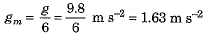Due to this very reason weight of an object on moon will be 1/6th of its weight on the earth.

Question for Chapter Notes: Gravitation
Try yourself:Question: Which of the following statements about weight is true?

## Thrust and Pressure

The normal force acting on a surface, due to the weight of an object placed on the surface, is called ‘thrust’. As thrust is a sort of force hence its SI unit is “a newton” (N).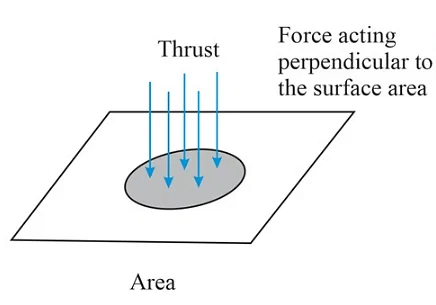Thrust

• The thrust on unit surface area is called pressure.
Pressure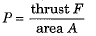Thus, pressure on a given object is the normal force acting on its surface per unit surface area.
SI unit of pressure is N m-2 but it is also called pascal and denoted by symbol Pa.
∴ 1 pascal (1 Pa) = 1 N m-2
• Same force acting on a smaller area exerts a larger pressure. It is due to this reason that a nail or a pin has a pointed tip and knives have sharp edges.
• Given force acting on a larger area exerts a smaller pressure. It is due to this reason that foundations of houses are made broad, base of dams is made broad, sleepers are laid below the railway line and so on.

### Pressure in Fluids

Fluid is that state of matter which can flow. All liquids and gases are fluids.Pressure in Fluids

• As fluids have weight, they exert pressure on the base and the walls of the container in which they are kept.
• In a fluid, pressure applied at any one place is transmitted equally in all directions.

### Buoyancy

• Whenever an object is immersed in a fluid, it experiences a force in the vertically upward direction due to that fluid. This force due to a fluid acting in upward direction  is called ‘force of buoyancy’ or ‘buoyant force or up thrust.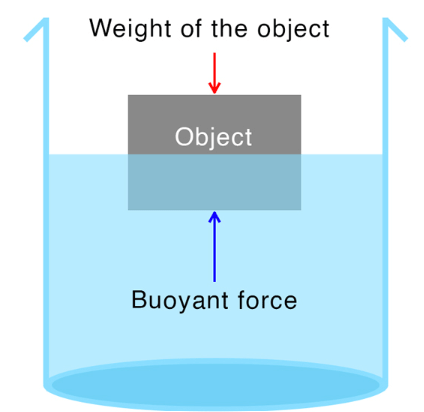Buoyancy

• Magnitude of force of buoyancy acting on an object depends upon
(i) the volume of an object immersed into the fluid, and
(ii) the density of the fluid. Due to force of buoyancy the net weight of an object becomes less than its normal weight.

Question for Chapter Notes: Gravitation
Try yourself:
What is the SI unit of weight?

### Why Objects Float Or Sink When Placed On The Surface Of Water?

The ability of an object to float or sink when placed on the surface of water is determined by its density and the density of the water. Density is a measure of how much mass is contained in a given volume.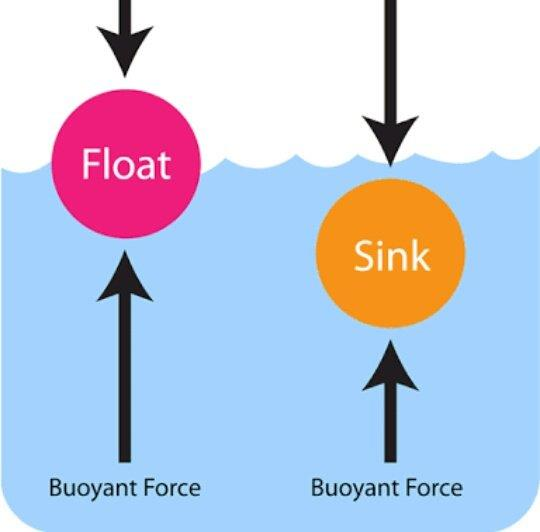Floating and Sinking on Surface of WaterWhen an object is placed in water, it experiences two main forces: buoyancy and gravity.

• Buoyancy is the upward force exerted on an object immersed in a fluid (in this case, water). It is caused by the difference in pressure between the top and bottom of the object. The greater the volume of water displaced by the object, the greater the buoyant force. According to Archimedes' principle, an object will experience an upward buoyant force equal to the weight of the water it displaces. If the buoyant force is greater than the object's weight, it will float. If the buoyant force is less than the object's weight, it will sink.
• Gravity, on the other hand, is the downward force pulling the object toward the center of the Earth. The weight of the object depends on its mass and the acceleration due to gravity. If the weight of the object is greater than the buoyant force, it will sink. If the weight is less than the buoyant force, it will float.

In summary, whether an object floats or sinks in water depends on the comparison between its weight and the buoyant force exerted by the water. If the object's weight is greater, it will sink. If the buoyant force is greater, it will float.

## Archimedes’ Principle

A Greek scientist Archimedes discovered a principle regarding buoyant force (or the loss in weight of an object when immersed in a fluid).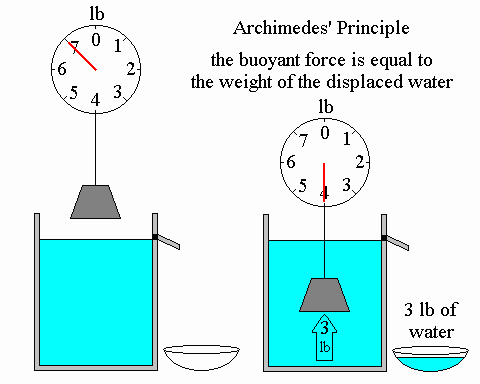Archimedes' Principle

• According to Archimedes’ principle “whenever an object is immersed fully or partially in a fluid, it experiences an upward force that is equal to the weight of the fluid displaced by it”.
• Alternately, according to Archimedes’ principle “there is always a loss (decrease) in weight of an object when it is immersed fully or partially in a fluid and the loss in weight is equal to the weight of the fluid displaced by the immersed part of given object”.
• Archimedes’ principle has many applications. Design of ships, submarines, lactometer and hydrometer are some of the applications of Archimedes’ principle.

Question for Chapter Notes: Gravitation
Try yourself:Archimedes' principle states that:

### Relative Density (Old Syllabus)

• Density of a substance is defined as its mass per unit volume. Its SI unit is kg m-3. However, sometimes unit g cm-3 is also used for density. It is found that 1 g cm-3 = 1000 kg m-3.
• Relative density of a substance is the ratio of its density to the density of water.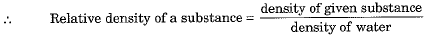Relative density is a unit-less quantity.
• We know that an iron nail immersed in water sinks but a wooden piece remains floating on water. It is found that objects having density less than that of the liquid, in which they are immersed, float on the surface of fluid. On the other hand, if density of the object is more than the density of the fluid in which it is immersed then the object will sink in that fluid.
The document Gravitation Chapter Notes | Science Class 9 is a part of the Class 9 Course Science Class 9.
All you need of Class 9 at this link: Class 9

## Science Class 9

66 videos|355 docs|97 tests

## FAQs on Gravitation Chapter Notes - Science Class 9

 1. What is gravitation?Ans. Gravitation is the natural phenomenon by which all objects with mass or energy are brought toward one another. It is the force that attracts two objects towards each other.
 2. What is the difference between free fall and gravity?Ans. Free fall refers to the motion of an object under the influence of gravity alone, with no other forces acting upon it. Gravity, on the other hand, is the force that causes objects to fall towards the Earth or any other celestial body.
 3. How is mass related to gravitation?Ans. Mass is a measure of the amount of matter in an object, while gravitation is the force that attracts objects towards each other. The greater the mass of an object, the stronger its gravitational pull.
 4. What is the difference between weight and mass?Ans. Weight is the force of gravity acting on an object, while mass is the amount of matter in an object. Weight depends on the mass of an object and the strength of the gravitational field it is in.
 5. How does Archimedes' Principle relate to gravitation?Ans. Archimedes' Principle states that an object immersed in a fluid experiences an upward buoyant force equal to the weight of the fluid it displaces. This principle is related to gravitation as it helps explain why objects float or sink in fluids, based on their density and the gravitational forces acting upon them.

## Science Class 9

66 videos|355 docs|97 testsExplore Courses for Class 9 examSignup to see your scores go up within 7 days! Learn & Practice with 1000+ FREE Notes, Videos & Tests.
10M+ students study on EduRev
Track your progress, build streaks, highlight & save important lessons and more!
Related Searches

,

,

,

,

,

,

,

,

,

,

,

,

,

,

,

,

,

,

,

,

,

;